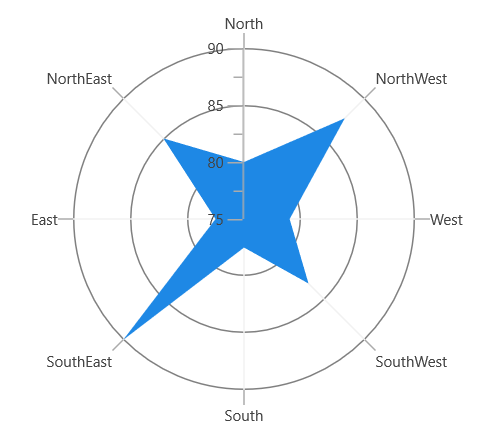# Radar and Polar in WinUI Chart

27 May 20226 minutes to read

`RadarSeries` represents a collection of data displayed by quantitative variables represented by axes starting from the same point. The relative position and angle of the axes are not uniform.

The following code example illustrates the use of radar series:

``````<chart:RadarSeries ItemsSource="{Binding PlantDetails}"

XBindingPath="Direction"

YBindingPath="Tree">

``````RadarSeries series = new RadarSeries()
{

ItemsSource = new ViewModel().PlantDetails,

XBindingPath = "Direction",

YBindingPath = "Tree",

};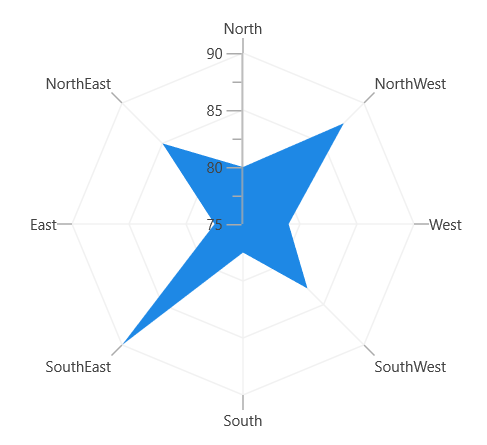## Polar

`PolarSeries` displays data points that are grouped by category on a 360-degree circle. The following code example shows how to use polar series.

``````<chart:PolarSeries

ItemsSource="{Binding PlantDetails}"

XBindingPath="Direction"

YBindingPath="Tree" />``````
``````PolarSeries series = new PolarSeries()
{

ItemsSource = new ViewModel().PlantDetails,

XBindingPath = "Direction",

YBindingPath = "Tree",

};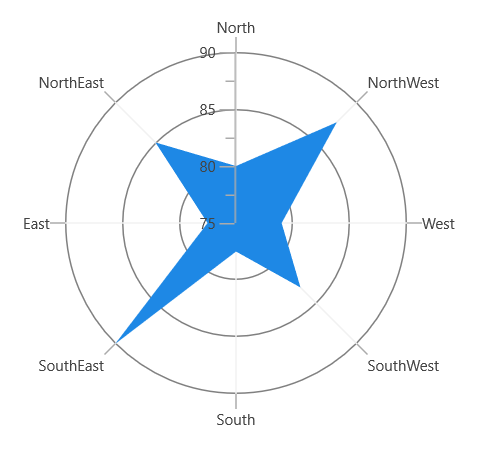The Radar and Polar charts have the following properties in common:

### IsClosed

This property is used to draw the closed path as follows.

``````<chart:PolarSeries x:Name="series1"

ItemsSource="{Binding PlantDetails}"

Label="Amount Spent" DrawType="Line" IsClosed="False"

XBindingPath="Direction" YBindingPath="Tree"

StrokeThickness="2" />``````
``````PolarSeries series = new PolarSeries()
{

ItemsSource = new ViewModel().PlantDetails,

XBindingPath = "Direction",

YBindingPath = "Tree",

IsClosed = false,

DrawType = ChartSeriesDrawType.Line,

Label = "Amount Spent",

StrokeThickness = 2,

};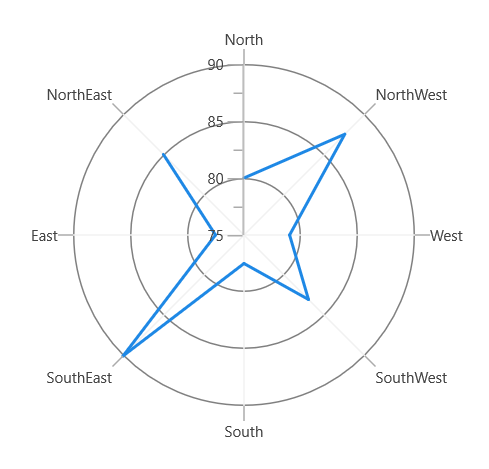### DrawType

This property defines type of curve, whether it is `Line` or `Area`.

DrawType as Area

``````<chart:PolarSeries x:Name="series1"

ItemsSource="{Binding PlantDetails}"

DrawType="Area" IsClosed="True"

XBindingPath="Direction" YBindingPath="Tree" />``````
``````PolarSeries series = new PolarSeries()
{

ItemsSource = new ViewModel().PlantDetails,

XBindingPath = "Direction",

YBindingPath = "Tree",

IsClosed = True,

DrawType = ChartSeriesDrawType.Area,

};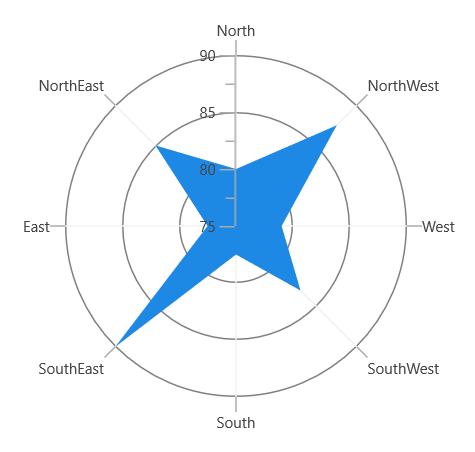DrawType as Line

``````<chart:PolarSeries

ItemsSource="{Binding PlantDetails}"

DrawType="Line" IsClosed="True"

XBindingPath="Direction" YBindingPath="Tree"

StrokeThickness="2" />``````
``````PolarSeries series = new PolarSeries()
{

ItemsSource = new ViewModel().PlantDetails,

XBindingPath = "Direction",

YBindingPath = "Tree",

IsClosed = True,

DrawType = ChartSeriesDrawType.Line,

StrokeThickness = 2,

};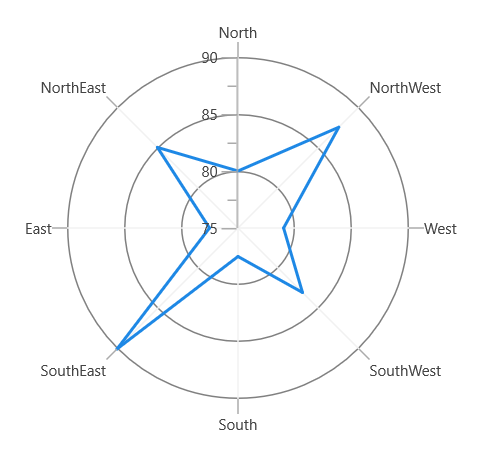### Polar angle

`Chart axis` provides support to render polar and radar series on 0,90,180 and 270 degrees. It can be achieved by its `PolarAngle` property. The ‘PolarAngle’ is a type of ‘ChartPolarAngle,’ and its default value is ‘Rotate270.’ Other supported values of ‘PolarAngle’ include ‘Rotate0,’ ‘Rotate90,’ and ‘Rotate180.’ Both the primary and secondary axes can be rotated individually based on their `PolarAngle` value.

Rotate0

The following sample explains how the axes of the series have been rotated when the `PolarAngle` value is `Rotate0`.

``````<chart:SfChart.PrimaryAxis>

<chart:CategoryAxis PolarAngle="Rotate0"/>

</chart:SfChart.PrimaryAxis>

<chart:SfChart.SecondaryAxis>

<chart:NumericalAxis PolarAngle="Rotate0"/>

</chart:SfChart.SecondaryAxis>``````
``````chart.PrimaryAxis = new CategoryAxis()
{

PolarAngle = ChartPolarAngle.Rotate0

};

chart.SecondaryAxis = new NumericalAxis()
{

PolarAngle = ChartPolarAngle.Rotate0

};``````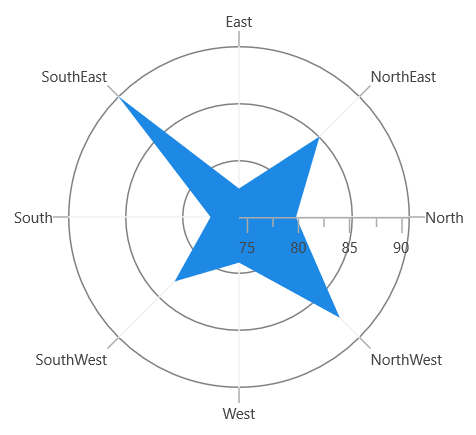Rotate90

The following sample explains how the axes of the series have been rotated when the `PolarAngle` value is `Rotate90`.

``````<chart:SfChart.PrimaryAxis>

<chart:CategoryAxis PolarAngle="Rotate90"/>

</chart:SfChart.PrimaryAxis>

<chart:SfChart.SecondaryAxis>

<chart:NumericalAxis PolarAngle="Rotate90"/>

</chart:SfChart.SecondaryAxis>``````
``````chart.PrimaryAxis = new CategoryAxis()
{

PolarAngle = ChartPolarAngle.Rotate90

};

chart.SecondaryAxis = new NumericalAxis()
{

PolarAngle = ChartPolarAngle.Rotate90

};``````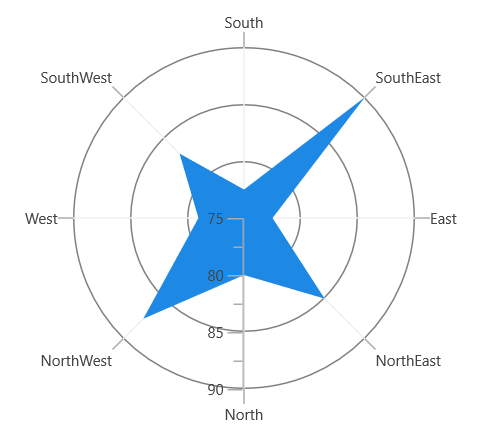Rotate180

The following sample explains how the axes of the series have been rotated when the `PolarAngle` value is `Rotate180`.

``````<chart:SfChart.PrimaryAxis>

<chart:CategoryAxis PolarAngle="Rotate180"/>

</chart:SfChart.PrimaryAxis>

<chart:SfChart.SecondaryAxis>

<chart:NumericalAxis PolarAngle="Rotate180"/>

</chart:SfChart.SecondaryAxis>``````
``````chart.PrimaryAxis = new CategoryAxis()
{

PolarAngle = ChartPolarAngle.Rotate180

};

chart.SecondaryAxis = new NumericalAxis()
{

PolarAngle = ChartPolarAngle.Rotate180

};``````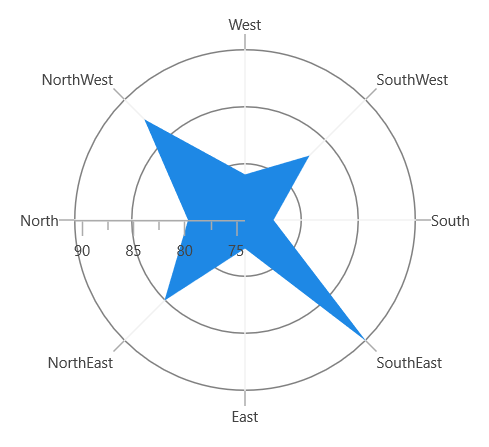Rotate270

The following sample explains how the axes of the series have been rotated when the `PolarAngle` value is `Rotate270`.

``````<chart:SfChart.PrimaryAxis>

<chart:CategoryAxis PolarAngle="Rotate270"/>

</chart:SfChart.PrimaryAxis>

<chart:SfChart.SecondaryAxis>

<chart:NumericalAxis PolarAngle="Rotate270"/>

</chart:SfChart.SecondaryAxis>``````
``````chart.PrimaryAxis = new CategoryAxis()
{

PolarAngle = ChartPolarAngle.Rotate270

};

chart.SecondaryAxis = new NumericalAxis()
{

PolarAngle = ChartPolarAngle.Rotate270

};``````